# Predicting Students' Dropout and Academic Success via Classification

Betul Mescioglu
import pandas as pd
from sklearn.model_selection import train_test_split
from sklearn.neighbors import KNeighborsClassifier
from sklearn.preprocessing import StandardScaler
import numpy as np
from sklearn.model_selection import cross_val_score, KFold
from sklearn.metrics import classification_report, confusion_matrix
from sklearn.linear_model import LogisticRegression
from sklearn.model_selection import GridSearchCV
from sklearn.model_selection import RandomizedSearchCV
from sklearn.inspection import permutation_importance
from sklearn.svm import SVC
from sklearn.metrics import accuracy_score
from sklearn.linear_model import SGDClassifier
from sklearn.model_selection import cross_validate
from sklearn.feature_selection import RFE
from sklearn.ensemble import RandomForestClassifier
import seaborn as sns

import warnings
warnings.filterwarnings("ignore")
C:\Users\h\anaconda3\lib\site-packages\pandas\core\computation\expressions.py:20: UserWarning: Pandas requires version '2.7.3' or newer of 'numexpr' (version '2.7.1' currently installed).
from pandas.core.computation.check import NUMEXPR_INSTALLED


## Understanding and Pre-Processing the Data:

The dataset was obtained from a higher education institution in Portugal by M.V.Martins, D. Tolledo, J. Machado, L. M.T. Baptista, V.Realinho, who also wrote the paper “Early prediction of student’s performance in higher education: a case study”(2021) using this dataset. The funding was provided by the program SATDAP - Capacitação da Administração Pública. The purpose of creating this dataset was to contribute to the reduction of academic dropout and failure in higher education by using machine learning techniques. The dataset includes information on academic path, demographics, and socioeconomic factors related to the students who enrolled in undergraduate degrees, such as education, nursing, and journalism at the time, as well as the students’ academic performance at the end of the first and second semesters. The ‘Target’ values were ‘dropout’, ‘enrolled’ and ‘graduate’. By using the provided features and employing classification techniques, I classified the data into these three categories. This is important as the institution can identify the students who are on the “dropout path” and intervene earlier to ensure they have all the necessary means to continue their education.

The dataset consists of 36 features, a “Target” column and 4424 student records as can be seen in Table 1 in Appendix. Features describe each student in terms of their demographic, socioeconomic status, and academic standing. Some of the demographic features are nationality, gender, age, and whether they are international student or not. Some of the socioeconomic features are marital status, father’s occupation, mother’s occupation and whether tuition fees are up to date or not. Some of the academic standing features are grade of previous degree, admission grade and grades of the first and second semesters and how many curricular credits the student acquired. All the values in the dataset are numeric except the “Target” column where values were labeled as ‘Dropout’, ‘Enrolled’, and ‘Graduate’. I used all the features in my analysis.

The dataset can be found at https://archive.ics.uci.edu/dataset/697/predict+students+dropout+and+academic+success

## Methodology:

Before applying any classification model, I needed to preprocess the data. The authors who provided the data performed a rigorous preprocessing to handle data from anomalies, unexplainable outliers, and missing values. Hence, the only preprocessing steps that I performed involved encoding the "Target" values, as described earlier, scaling the data using a Standard Scaler and splitting the data into train, validation and test sets. Twenty percent of the data was set aside as the test set, twenty percent of the remaining data was set aside as the validation set, and the remaining part was used as the training set. Training set had 2831 instances, validation set had 708 instances and testing set had 885 instances. All hyperparameter tunings were carried out on the training data, and tested on the validation data. Finally, tuned models were applied to test set to measure the success of the model.

Four classification algorithms were applied; K Nearest Neighbors (KNN), Support Vector Machines (SVM), SGDClassifier (used as a Logistic Regression Classifier) and Random Forests. For each model, manual cross validation was performed and average and standard deviation of the scores were computed to observe whether overfitting occurred. Additionally, grid search cross validation was performed to obtain the best hyperparameters. Consequently, the models tuned with these best hyperparameters were applied on the test sets. Finally, as statistic measures, confusion matrices, classification reports and accuracy scores were printed for validation and test sets. Additionally, features that were used in decision making were plotted for each model.

df = pd.read_csv('data.csv', sep=';')
df.head()
Marital status Application mode Application order Course Daytime/evening attendance\t Previous qualification Previous qualification (grade) Nacionality Mother's qualification Father's qualification ... Curricular units 2nd sem (credited) Curricular units 2nd sem (enrolled) Curricular units 2nd sem (evaluations) Curricular units 2nd sem (approved) Curricular units 2nd sem (grade) Curricular units 2nd sem (without evaluations) Unemployment rate Inflation rate GDP Target
0 1 17 5 171 1 1 122.0 1 19 12 ... 0 0 0 0 0.000000 0 10.8 1.4 1.74 Dropout
1 1 15 1 9254 1 1 160.0 1 1 3 ... 0 6 6 6 13.666667 0 13.9 -0.3 0.79 Graduate
2 1 1 5 9070 1 1 122.0 1 37 37 ... 0 6 0 0 0.000000 0 10.8 1.4 1.74 Dropout
3 1 17 2 9773 1 1 122.0 1 38 37 ... 0 6 10 5 12.400000 0 9.4 -0.8 -3.12 Graduate
4 2 39 1 8014 0 1 100.0 1 37 38 ... 0 6 6 6 13.000000 0 13.9 -0.3 0.79 Graduate

5 rows × 37 columns

df.shape
(4424, 37)
df.Target.value_counts()
Graduate    2209
Dropout     1421
Enrolled     794
Name: Target, dtype: int64
df.info()
<class 'pandas.core.frame.DataFrame'>
RangeIndex: 4424 entries, 0 to 4423
Data columns (total 37 columns):
#   Column                                          Non-Null Count  Dtype
---  ------                                          --------------  -----
0   Marital status                                  4424 non-null   int64
1   Application mode                                4424 non-null   int64
2   Application order                               4424 non-null   int64
3   Course                                          4424 non-null   int64
4   Daytime/evening attendance	                     4424 non-null   int64
5   Previous qualification                          4424 non-null   int64
6   Previous qualification (grade)                  4424 non-null   float64
7   Nacionality                                     4424 non-null   int64
8   Mother's qualification                          4424 non-null   int64
9   Father's qualification                          4424 non-null   int64
10  Mother's occupation                             4424 non-null   int64
11  Father's occupation                             4424 non-null   int64
13  Displaced                                       4424 non-null   int64
14  Educational special needs                       4424 non-null   int64
15  Debtor                                          4424 non-null   int64
16  Tuition fees up to date                         4424 non-null   int64
17  Gender                                          4424 non-null   int64
18  Scholarship holder                              4424 non-null   int64
19  Age at enrollment                               4424 non-null   int64
20  International                                   4424 non-null   int64
21  Curricular units 1st sem (credited)             4424 non-null   int64
22  Curricular units 1st sem (enrolled)             4424 non-null   int64
23  Curricular units 1st sem (evaluations)          4424 non-null   int64
24  Curricular units 1st sem (approved)             4424 non-null   int64
25  Curricular units 1st sem (grade)                4424 non-null   float64
26  Curricular units 1st sem (without evaluations)  4424 non-null   int64
27  Curricular units 2nd sem (credited)             4424 non-null   int64
28  Curricular units 2nd sem (enrolled)             4424 non-null   int64
29  Curricular units 2nd sem (evaluations)          4424 non-null   int64
30  Curricular units 2nd sem (approved)             4424 non-null   int64
31  Curricular units 2nd sem (grade)                4424 non-null   float64
32  Curricular units 2nd sem (without evaluations)  4424 non-null   int64
33  Unemployment rate                               4424 non-null   float64
34  Inflation rate                                  4424 non-null   float64
35  GDP                                             4424 non-null   float64
36  Target                                          4424 non-null   object
dtypes: float64(7), int64(29), object(1)
memory usage: 1.2+ MB

df.isna().sum()
Marital status                                    0
Application mode                                  0
Application order                                 0
Course                                            0
Daytime/evening attendance\t                      0
Previous qualification                            0
Nacionality                                       0
Mother's qualification                            0
Father's qualification                            0
Mother's occupation                               0
Father's occupation                               0
Displaced                                         0
Educational special needs                         0
Debtor                                            0
Tuition fees up to date                           0
Gender                                            0
Scholarship holder                                0
Age at enrollment                                 0
International                                     0
Curricular units 1st sem (credited)               0
Curricular units 1st sem (enrolled)               0
Curricular units 1st sem (evaluations)            0
Curricular units 1st sem (approved)               0
Curricular units 1st sem (grade)                  0
Curricular units 1st sem (without evaluations)    0
Curricular units 2nd sem (credited)               0
Curricular units 2nd sem (enrolled)               0
Curricular units 2nd sem (evaluations)            0
Curricular units 2nd sem (approved)               0
Curricular units 2nd sem (grade)                  0
Curricular units 2nd sem (without evaluations)    0
Unemployment rate                                 0
Inflation rate                                    0
GDP                                               0
Target                                            0
dtype: int64
#Convert Target variables to numbers
def label(row):
return 2
elif row =='Enrolled':
return 1
else:
return 0
df['Target'] = df['Target'].apply(label)
df['Target'].value_counts()
2    2209
0    1421
1     794
Name: Target, dtype: int64

The frequency distribution of all the features and the “Target” column can be seen in the following figure. We observe that most of the features are discrete, and continuous features, such as grades columns exhibit a Gaussian distribution pattern. “Target” values are encoded as “Graduate” => 2, “Enrolled”=> 1, and “Dropout”=> 0. The graduation rate among students is nearly three times higher than the enrollment rate and almost twice as high as the dropout rate.

import matplotlib.pyplot as  plt
df.hist(bins=50, figsize=(20,15))
plt.tight_layout()
plt.show()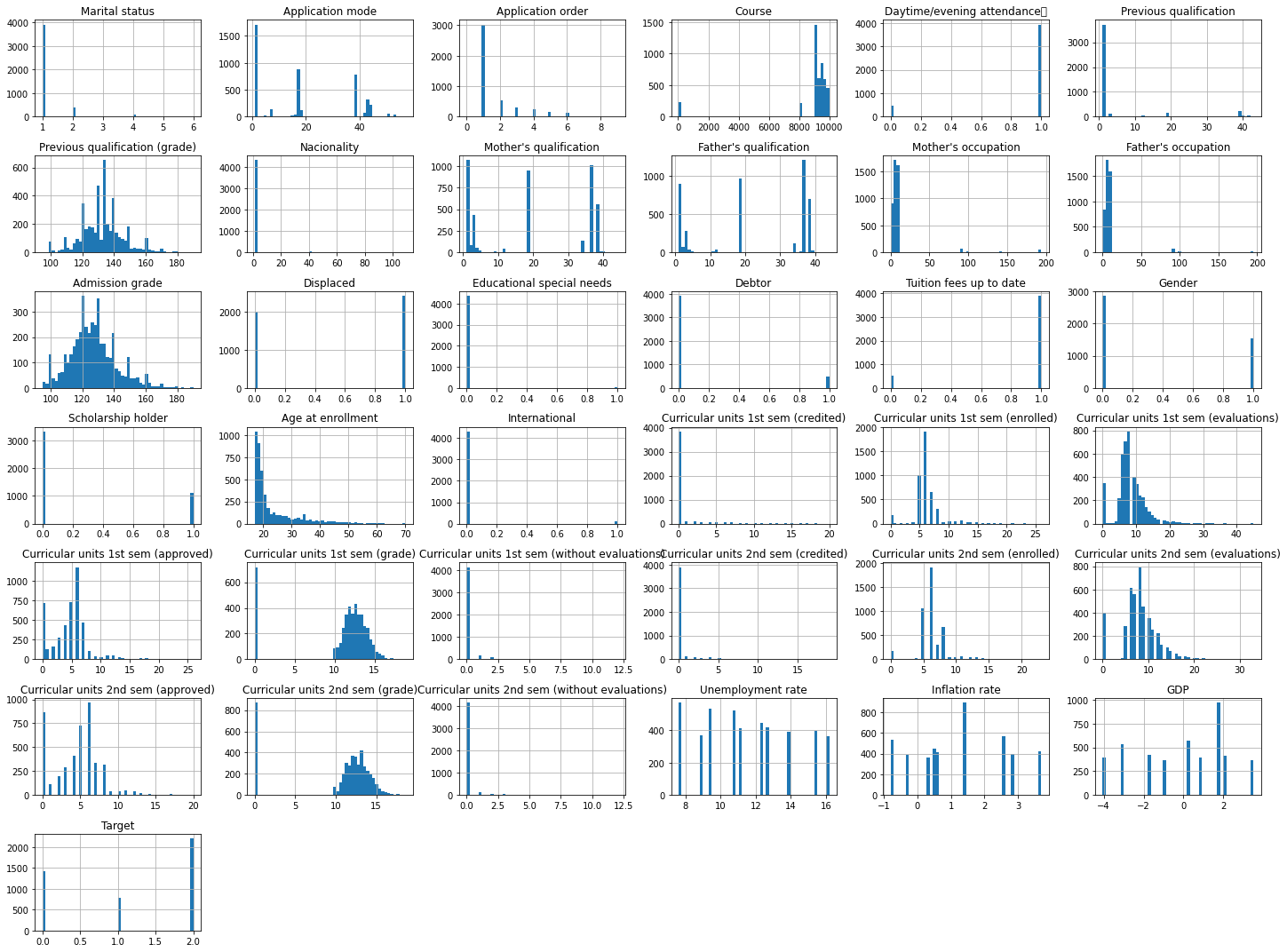Columns that are highly correlated with Target can be seen below.The columns that exhibit high correlation with “Target” column include grades and credits students acquired in the second and first semesters, tuition and scholarship information among others. Second semester’s correlation is higher than others, as finishing the second the semester is a good indicator of how invested students are in the program.

corr_df = pd.DataFrame(df.corr()['Target'].abs().sort_values(ascending=False)[:16])[1:]
corr_df.index
corr_df
Target
Curricular units 2nd sem (approved) 0.624157
Curricular units 2nd sem (grade) 0.566827
Curricular units 1st sem (approved) 0.529123
Curricular units 1st sem (grade) 0.485207
Tuition fees up to date 0.409827
Scholarship holder 0.297595
Age at enrollment 0.243438
Debtor 0.240999
Gender 0.229270
Application mode 0.221747
Curricular units 2nd sem (enrolled) 0.175847
Curricular units 1st sem (enrolled) 0.155974
Displaced 0.113986

### Scaling the Dataset:

SS = StandardScaler()
df_scaled = pd.DataFrame(SS.fit_transform(df.iloc[:,:-1]), columns=df.columns[:-1])
df_scaled['Target'] = df['Target']
df_scaled.head()
Marital status Application mode Application order Course Daytime/evening attendance\t Previous qualification Previous qualification (grade) Nacionality Mother's qualification Father's qualification ... Curricular units 2nd sem (credited) Curricular units 2nd sem (enrolled) Curricular units 2nd sem (evaluations) Curricular units 2nd sem (approved) Curricular units 2nd sem (grade) Curricular units 2nd sem (without evaluations) Unemployment rate Inflation rate GDP Target
0 -0.294829 -0.095470 2.490896 -4.209520 0.350082 -0.35023 -0.804841 -0.126298 -0.036018 -0.669778 ... -0.282442 -2.838337 -2.042630 -1.471527 -1.963489 -0.199441 -0.287638 0.124386 0.765761 0
1 -0.294829 -0.209869 -0.554068 0.192580 0.350082 -0.35023 2.076819 -0.126298 -1.189759 -1.256427 ... -0.282442 -0.105726 -0.522682 0.518904 0.659562 -0.199441 0.876222 -1.105222 0.347199 2
2 -0.294829 -1.010660 2.490896 0.103404 0.350082 -0.35023 -0.804841 -0.126298 1.117723 0.959802 ... -0.282442 -0.105726 -2.042630 -1.471527 -1.963489 -0.199441 -0.287638 0.124386 0.765761 0
3 -0.294829 -0.095470 0.207173 0.444115 0.350082 -0.35023 -0.804841 -0.126298 1.181819 0.959802 ... -0.282442 -0.105726 0.490616 0.187165 0.416450 -0.199441 -0.813253 -1.466871 -1.375511 2
4 1.356212 1.162916 -0.554068 -0.408389 -2.856470 -0.35023 -2.473171 -0.126298 1.117723 1.024985 ... -0.282442 -0.105726 -0.522682 0.518904 0.531608 -0.199441 0.876222 -1.105222 0.347199 2

5 rows × 37 columns

### Splitting the Dataset:

X and y are what is left after removing X_test and y_test. X and y are divided into train and validation sets.

X_df = df_scaled.drop('Target', axis=1).values
y_df = df['Target'].values
X, X_test, y, y_test =train_test_split(X_df, y_df, test_size=0.2, random_state=21, stratify=y_df)
X_train, X_val, y_train, y_val = train_test_split(X, y, test_size=0.2, random_state=21, stratify=y)
print('X_train shape: ', len(X_train))
print('y_train shape:', len(y_train))
print('X_val shape: ', len(X_val))
print('y_val shape:', len(y_val))
print('X_test shape: ', len(X_test))
print('y_test shape: ', len(y_test))
X_train shape:  2831
y_train shape: 2831
X_val shape:  708
y_val shape: 708
X_test shape:  885
y_test shape:  885


# Applying KNearestNeighbors:

#### Hyperparameter Tuning:

train_acc_knn = {}
test_acc_knn = {}
neighbors = np.arange(1, 26)
for neighbor in neighbors:
knn = KNeighborsClassifier(n_neighbors=neighbor)
knn.fit(X_train, y_train)
train_acc_knn[neighbor] = knn.score(X_train, y_train)
test_acc_knn[neighbor] = knn.score(X_val, y_val)

plt.title("KNN: Varying Number of Neighbors")

# Plot training accuracies
plt.plot(neighbors, train_acc_knn.values(), label="Training Accuracy")

# Plot test accuracies
plt.plot(neighbors, test_acc_knn.values(), label="Testing Accuracy")

plt.legend()
plt.xlabel("Number of Neighbors")
plt.ylabel("Accuracy")

# Display the plot
plt.show()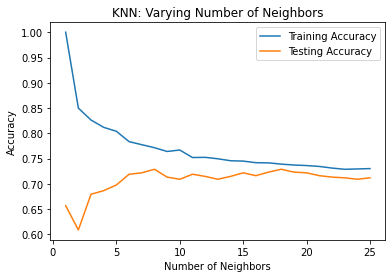print("Number of neighbors that results in the highest accuracy: {}".format(max(test_acc_knn, key=test_acc_knn.get)))
Number of neighbors that results in the highest accuracy: 8


#### Cross Validation:

##### Manual Cross Validation:
knn_manual = KNeighborsClassifier()
kf = KFold(n_splits=6, shuffle=True, random_state=42)
cv_results = cross_val_score(knn_manual, X, y, cv=kf)
cv_results
array([0.68135593, 0.68135593, 0.70677966, 0.70677966, 0.70677966,
0.68590832])
print('Mean of cross val. results is {}, standard variation is {}'.format(np.mean(cv_results).round(4), np.std(cv_results).round(4)))
Mean of cross val. results is 0.6948, standard variation is 0.012

print('95% confidence interval is {}'.format(np.quantile(cv_results, [0.025, 0.975]).round(4)))
95% confidence interval is [0.6814 0.7068]

knn_tuned = KNeighborsClassifier(n_neighbors=18)
knn_tuned.fit(X_train, y_train)
knn_tuned.score(X_val,y_val)

0.7288135593220338
kf = KFold(n_splits=5, shuffle=True, random_state=42)
param_grid = {'n_neighbors':[8,11, 15, 18], 'metric':['euclidean', 'manhattan']}
knn = KNeighborsClassifier()
knn_gs = GridSearchCV(knn, param_grid, cv=kf)
knn_gs.fit(X_train, y_train)
print(knn_gs.best_params_, knn_gs.best_score_)

{'metric': 'manhattan', 'n_neighbors': 18} 0.7276646661805672

knn_random = RandomizedSearchCV(knn, param_grid, cv=kf, n_iter=2)
knn_random.fit(X_train, y_train)
print(knn_random.best_params_, knn_random.best_score_)
{'n_neighbors': 11, 'metric': 'manhattan'} 0.7262474993923757


Manual and grid search both give the validation accuracy at 73%. We will use the parameters found by grid search.

### Validation Results Using the Tuned KNN:

knn_tuned_grid = KNeighborsClassifier(n_neighbors=18, metric='manhattan')
knn_tuned_grid.fit(X_train, y_train)
knn_tuned_grid.score(X_train, y_train).round(4)
0.7549
knn_tuned_grid = KNeighborsClassifier(n_neighbors=18, metric='manhattan')
knn_tuned_grid.fit(X_train, y_train)
knn_tuned_grid.score(X_val, y_val).round(4)
0.7161

### Finding the most Relevant Features:

result = permutation_importance(knn_tuned_grid, X_val, y_val, n_repeats=10, random_state=42)
importances = result.importances_mean.round(5)
important_feat_knn = pd.DataFrame(importances, df_scaled.columns[:-1], columns=['importance'])
important_feat_knn.sort_values(by='importance', ascending=False)
importance
Curricular units 2nd sem (approved) 0.02062
Tuition fees up to date 0.01412
Debtor 0.00847
Curricular units 2nd sem (evaluations) 0.00593
Scholarship holder 0.00551
Curricular units 1st sem (approved) 0.00395
Curricular units 2nd sem (grade) 0.00353
Curricular units 2nd sem (credited) 0.00212
Curricular units 2nd sem (without evaluations) 0.00155
Application mode 0.00085
Mother's occupation 0.00042
Marital status 0.00028
Educational special needs 0.00014
Curricular units 1st sem (credited) 0.00014
Curricular units 1st sem (without evaluations) -0.00056
Curricular units 1st sem (evaluations) -0.00071
Father's occupation -0.00085
Curricular units 1st sem (grade) -0.00127
Inflation rate -0.00184
Displaced -0.00212
Age at enrollment -0.00254
Nacionality -0.00282
International -0.00297
Mother's qualification -0.00381
Daytime/evening attendance\t -0.00395
Application order -0.00395
Father's qualification -0.00438
GDP -0.00452
Previous qualification -0.00480
Curricular units 1st sem (enrolled) -0.00537
Curricular units 2nd sem (enrolled) -0.00579
Unemployment rate -0.00692
Gender -0.00706
Course -0.00763
important_feat_knn.plot(kind='barh', figsize=(12,10))
<AxesSubplot: >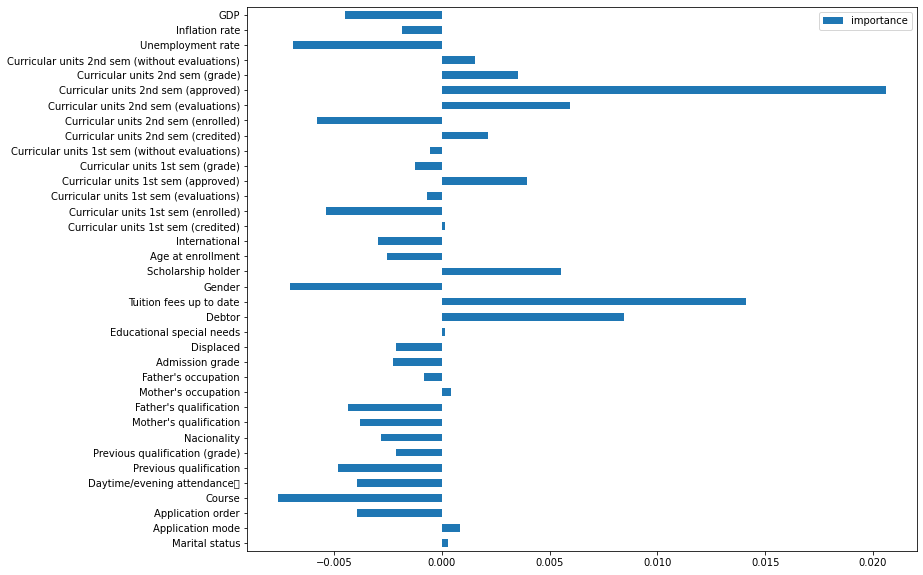### Applying tuned KNN to Unseen Data:

predicted = knn_tuned_grid.predict(X_test)
print(confusion_matrix(y_test, predicted))

print(classification_report(y_test, predicted))
print(accuracy_score(y_test, predicted).round(4))
sns.heatmap(confusion_matrix(y_test, predicted)/np.sum(confusion_matrix(y_test, predicted)), fmt='.2%',annot=True, cmap='Blues',cbar=False)
[[173  23  88]
[ 24  34 101]
[  6  11 425]]
precision    recall  f1-score   support

0       0.85      0.61      0.71       284
1       0.50      0.21      0.30       159
2       0.69      0.96      0.80       442

accuracy                           0.71       885
macro avg       0.68      0.59      0.60       885
weighted avg       0.71      0.71      0.68       885

0.7141

<AxesSubplot: >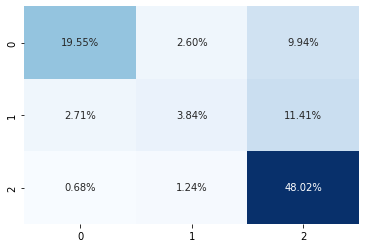## Applying Support Vector:

##### Manual Cross Validation:
svm = SVC()
cv_results = cross_val_score(svm, X,y, cv=kf)

print('Mean of cross validation results is {}, standard variation is {}'.format(np.mean(cv_results).round(4), np.std(cv_results).round(4)))
Mean of cross validation results is 0.7601, standard variation is 0.0049


Standard deviation is pretty low, all folds give similar results, therefore no overfitting.

print('95% confidence interval is {}'.format(np.quantile(cv_results, [0.025, 0.975]).round(4)))
95% confidence interval is [0.7531 0.7651]

svm =SVC()
params = {'C':[0.1, 1, 10], 'gamma':[0.00001, 0.0001, 0.001, 0.01, 0.1]}
searcher = GridSearchCV(svm, param_grid=params)
searcher.fit(X_train,y_train)
pred = searcher.predict(X_val)
accuracy_score(y_val, pred)
0.769774011299435
searcher.cv_results_
{'mean_fit_time': array([0.27595596, 0.55183644, 0.39222798, 0.2324347 , 0.30942407,
0.49004912, 0.29670954, 0.24211607, 0.16929913, 0.36431842,
0.25194054, 0.20276361, 0.16316075, 0.18086963, 0.42357111]),
'std_fit_time': array([0.03921296, 0.23337329, 0.09773754, 0.04982113, 0.03287002,
0.09668777, 0.06666036, 0.0211803 , 0.00628583, 0.13873721,
0.01022663, 0.0109675 , 0.00762966, 0.01226799, 0.02233543]),
'mean_score_time': array([0.15382695, 0.27605562, 0.19099441, 0.10318069, 0.12657542,
0.26565924, 0.13917098, 0.10622325, 0.08159723, 0.13483315,
0.11810298, 0.08284287, 0.07531404, 0.07977457, 0.12237706]),
'std_score_time': array([0.0348076 , 0.08090776, 0.07325376, 0.01809595, 0.02352529,
0.06000496, 0.02939992, 0.02223646, 0.00626639, 0.03520782,
0.01340029, 0.00863925, 0.00618585, 0.01213592, 0.01178965]),
'param_C': masked_array(data=[0.1, 0.1, 0.1, 0.1, 0.1, 1, 1, 1, 1, 1, 10, 10, 10, 10,
10],
mask=[False, False, False, False, False, False, False, False,
False, False, False, False, False, False, False],
fill_value='?',
dtype=object),
'param_gamma': masked_array(data=[1e-05, 0.0001, 0.001, 0.01, 0.1, 1e-05, 0.0001, 0.001,
0.01, 0.1, 1e-05, 0.0001, 0.001, 0.01, 0.1],
mask=[False, False, False, False, False, False, False, False,
False, False, False, False, False, False, False],
fill_value='?',
dtype=object),
'params': [{'C': 0.1, 'gamma': 1e-05},
{'C': 0.1, 'gamma': 0.0001},
{'C': 0.1, 'gamma': 0.001},
{'C': 0.1, 'gamma': 0.01},
{'C': 0.1, 'gamma': 0.1},
{'C': 1, 'gamma': 1e-05},
{'C': 1, 'gamma': 0.0001},
{'C': 1, 'gamma': 0.001},
{'C': 1, 'gamma': 0.01},
{'C': 1, 'gamma': 0.1},
{'C': 10, 'gamma': 1e-05},
{'C': 10, 'gamma': 0.0001},
{'C': 10, 'gamma': 0.001},
{'C': 10, 'gamma': 0.01},
{'C': 10, 'gamma': 0.1}],
'split0_test_score': array([0.49911817, 0.49911817, 0.63844797, 0.69664903, 0.63844797,
0.49911817, 0.64373898, 0.70017637, 0.7319224 , 0.72310406,
0.64373898, 0.70194004, 0.7319224 , 0.73544974, 0.70194004]),
'split1_test_score': array([0.49823322, 0.49823322, 0.66077739, 0.70848057, 0.66784452,
0.49823322, 0.66254417, 0.71378092, 0.77738516, 0.7614841 ,
0.66254417, 0.71378092, 0.76678445, 0.80388693, 0.74558304]),
'split2_test_score': array([0.49823322, 0.49823322, 0.65724382, 0.71378092, 0.65547703,
0.49823322, 0.6590106 , 0.72791519, 0.73674912, 0.7155477 ,
0.66254417, 0.72614841, 0.74381625, 0.74028269, 0.69257951]),
'split3_test_score': array([0.5       , 0.5       , 0.66254417, 0.73144876, 0.65724382,
0.5       , 0.66254417, 0.74381625, 0.78091873, 0.74911661,
0.66254417, 0.74381625, 0.77031802, 0.78975265, 0.72791519]),
'split4_test_score': array([0.5       , 0.5       , 0.66607774, 0.71201413, 0.66431095,
0.5       , 0.66607774, 0.72261484, 0.75441696, 0.7155477 ,
0.66431095, 0.72438163, 0.75265018, 0.75971731, 0.7155477 ]),
'mean_test_score': array([0.49911692, 0.49911692, 0.65701822, 0.71247468, 0.65666486,
0.49911692, 0.65878313, 0.72166072, 0.75627847, 0.73296003,
0.65913649, 0.72201345, 0.75309826, 0.76581786, 0.7167131 ]),
'std_test_score': array([0.00079013, 0.00079013, 0.00971234, 0.01121016, 0.01016906,
0.00079013, 0.00784704, 0.01452285, 0.02015298, 0.01885829,
0.00772911, 0.01391966, 0.01427309, 0.02695779, 0.01876695]),
'rank_test_score': array([13, 13, 11,  8, 12, 13, 10,  6,  2,  4,  9,  5,  3,  1,  7])}
gs_svm =pd.DataFrame(searcher.cv_results_['params'])
gs_svm['test_score'] = searcher.cv_results_['mean_test_score']
gs_svm
C gamma test_score
0 0.1 0.00001 0.499117
1 0.1 0.00010 0.499117
2 0.1 0.00100 0.657018
3 0.1 0.01000 0.712475
4 0.1 0.10000 0.656665
5 1.0 0.00001 0.499117
6 1.0 0.00010 0.658783
7 1.0 0.00100 0.721661
8 1.0 0.01000 0.756278
9 1.0 0.10000 0.732960
10 10.0 0.00001 0.659136
11 10.0 0.00010 0.722013
12 10.0 0.00100 0.753098
13 10.0 0.01000 0.765818
14 10.0 0.10000 0.716713
#Non interactive 3D
feat1 = gs_svm['C']
feat2 = gs_svm['gamma']
feat3 = gs_svm['test_score']

fig = plt.figure()

ax.scatter(feat1, feat2, feat3)

# Set labels for each axis
ax.set_xlabel('C')
ax.set_ylabel('gamma')
ax.set_zlabel('score')

# Show the plot
plt.title('Validation Results for Various C and gamma Values')
plt.show()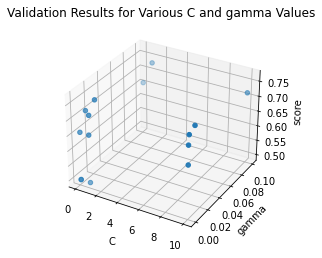#Plotting them in interactive form
import plotly.graph_objects as go

feat1 = gs_svm['C']
feat2 = gs_svm['gamma']
feat3 = gs_svm['test_score']

fig = go.Figure(data=[go.Scatter3d(x=feat1, y=feat2, z=feat3, mode='markers')])

fig.update_layout(scene=dict(xaxis_title='C', yaxis_title='gamma', zaxis_title='Score'), title='Validation Results for Various C and gamma Values')
fig.show()